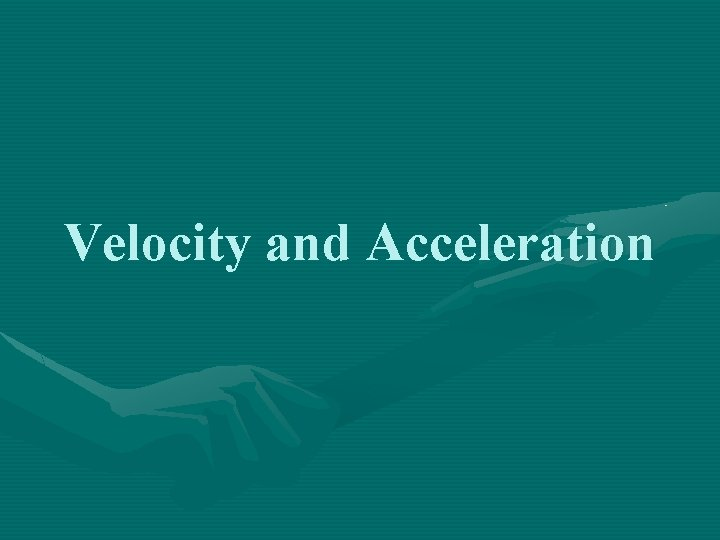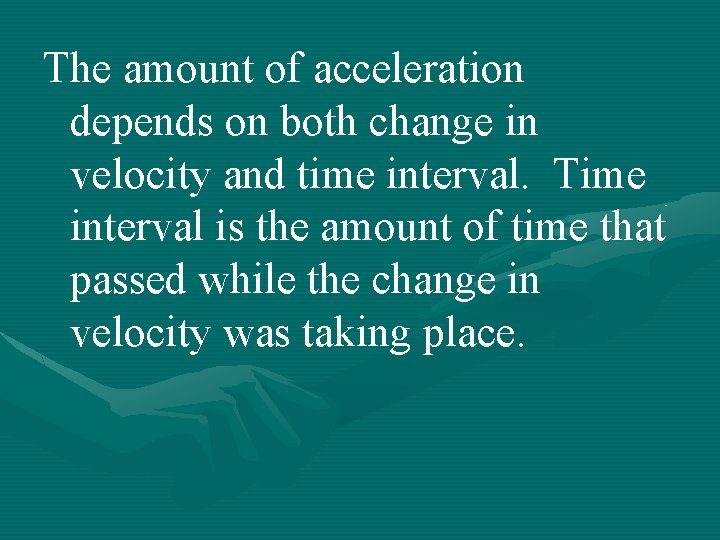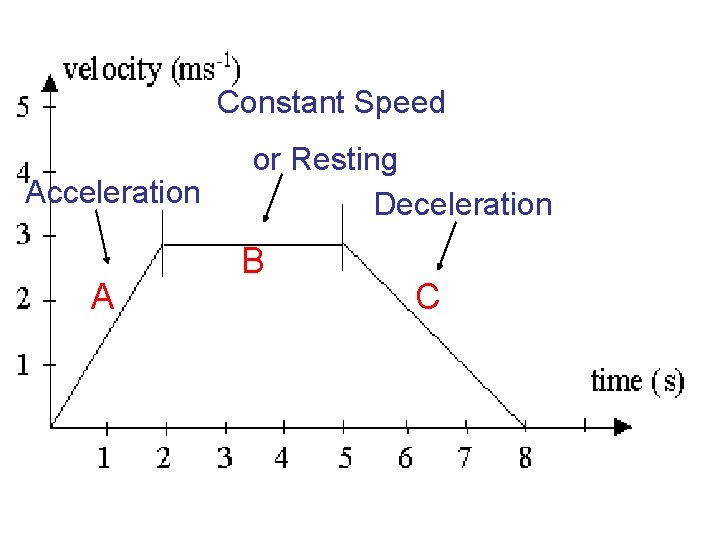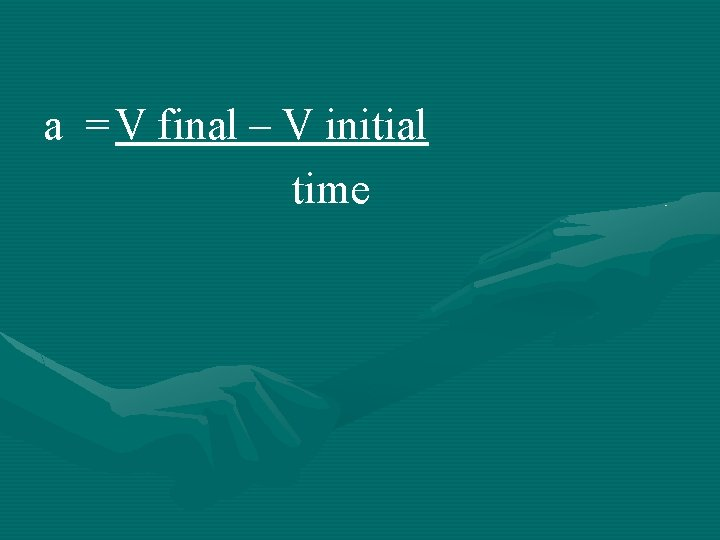# Velocity and Acceleration Velocity describes speed and the

• Slides: 10
Download presentationVelocity and Acceleration• Velocity- describes speed and the direction of an object. -Velocity can change. It can change even if the speed of the object remains constant. Ex: Car going around a curve. Speed is same but velocity changes (direction changes)• Acceleration- the rate of change of velocity. -Acceleration can occur through a change in speed or in direction.-If the acceleration is in the same direction as the velocity= you will speed up. Also called positive acceleration. -If the acceleration is in the different direction as the velocity = you will slow down. Also called negative acceleration or deceleration.The amount of acceleration depends on both change in velocity and time interval. Time interval is the amount of time that passed while the change in velocity was taking place.Constant Speed Acceleration A or Resting Deceleration B CCalculating Acceleration Variable Ending Unit a = acceleration m/s 2 or km/hr 2 t = time sec. or hr. v f = final velocity m/s or km/hr v i = start velocity m/s or km/hra = V final – V initial timeEx: A swimmer speeds up from 1. 1 m/s to 1. 3 m/s during the last 20 sec. of a workout. What is the swimmer’s acceleration during this time interval?Ex: While walking to school, you approach an intersection and slow down from 2 m/s to a stop in 3 sec. What was your acceleration during this time interval?